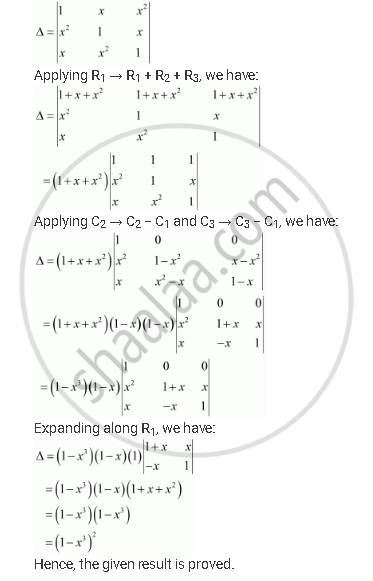Share

# By Using Properties of Determinants, Show That: |(1,X,Xsqrt2),(Xsqrt2,1,X),(X,Xsqrt2,1)| = (1-xcuberoot3)Sqrt2 - CBSE (Science) Class 12 - Mathematics

#### Question

By using properties of determinants, show that:

|(1,x,x^2),(x^2,1,x),(x,x^2,1)| = (1-x^3)^2

#### SolutionIs there an error in this question or solution?

#### APPEARS IN

Solution By Using Properties of Determinants, Show That: |(1,X,Xsqrt2),(Xsqrt2,1,X),(X,Xsqrt2,1)| = (1-xcuberoot3)Sqrt2 Concept: Properties of Determinants.
S